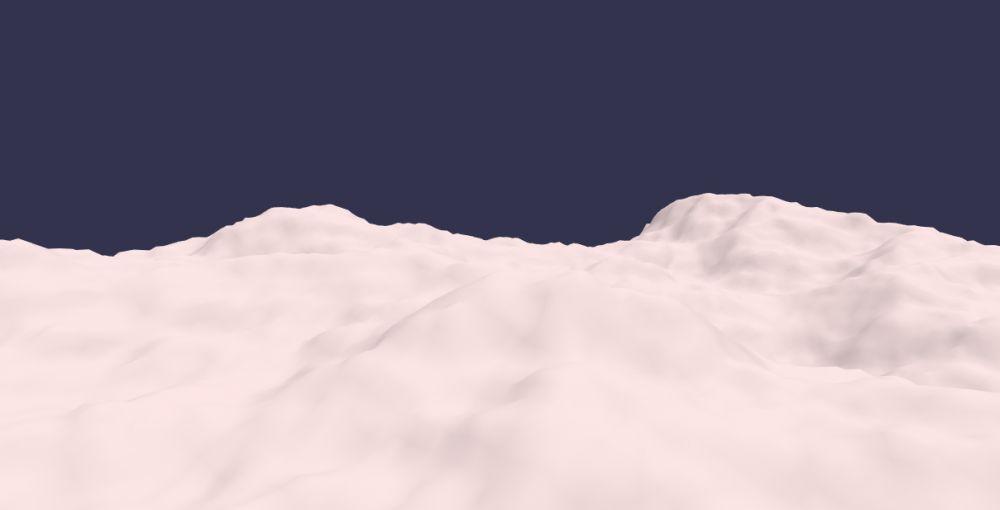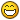# solved NullEngine and heightmaps/terrain

## Recommended Posts

What are some options for creating terrain from heightmaps via NullEngine? I'm trying to create a serverside terrain for collision checks in a multiplayer game.

The main issue is that Mesh.CreateGroundFromHeightMap involves loading an image and going through pixel data via canvas, none of which exists in node.

Some ideas:

Expose canvas + image api on the server: https://github.com/Automattic/node-canvas can provide Image and Canvas to NullEngine, though I've written a server side image processor recently that uses this tech and while it does work it isn't entirely straight forward because quite a few extra things need installed before it all works. I'm not sure how many babylon nullengine features use canvas/image, if its just this one then I doubt this route is worth it.

Just loading the image in node: a small patch function that detects if we're in node and loads an image and gets its pixels using regular node fs. I'm not 100% sure how to do this but it sounds possible. This would kinda fork the logic inside of Mesh.CreateGroundFromHeightMap unless it was exposed in a new way.

Go straight to the heightmap data and skip the images: https://doc.babylonjs.com/api/classes/babylon.vertexdata#creategroundfromheightmap - this does assume pixel data (UInt8 array of RGB? RGBA?) but has no dependency on canvas as far as I can tell. I don't understand the colorFilter (0.3, 0.59, 0.11) though, so someone would have to explain it to me.

In my case I actually generated my heightmaps in node to begin with, and then saved them as images purely as a visual tool to see how the maps would look. I happen to be using these debug images and loading them into babylon because it works automagically! I could certain just skip all this and save them in some other format, like a 1D array of UInt8s (though in this scenario it would be ideal to skip RGBA and just have raw height data). I could probably add this to babylon by following the existing example if this is a feature people want.

Once again I'm a total 3D noob, so if there is a much more trivial solution please let me know :D. I'm also willing to contribute, but I just don't want to make the wrong contribution.

##### Share on other sites

I tried the option of creating vertex data from a simple height map (as opposed to creating it from pixel data). I then used this code on both client and server and passed it a heightmap generated via a seed. It seems to work pretty well. For anyone interested the code is below.

The code is almost exactly the existing BJS height map code, except this one uses a 1D array of heights instead of pixels:

``````function vertexDataFromHeightMap(options) {
let indices = []
let positions = []
let normals = []
let uvs = []
let row
let col

for (row = 0; row <= options.subdivisions; row++) {
for (col = 0; col <= options.subdivisions; col++) {
let position = new BABYLON.Vector3(
(col * options.width) / options.subdivisions - (options.width / 2.0),
0,
((options.subdivisions - row) * options.height) / options.subdivisions - (options.height / 2.0)
)

let heightMapX = (((position.x + options.width / 2) / options.width) * (options.bufferWidth - 1)) | 0
let heightMapY = ((1.0 - (position.z + options.height / 2) / options.height) * (options.bufferHeight - 1)) | 0
let pos = (heightMapX + heightMapY * options.bufferWidth)
let height = options.buffer[pos] / 255
position.y = options.minHeight + (options.maxHeight - options.minHeight) * height
positions.push(position.x, position.y, position.z)
normals.push(0, 0, 0)
uvs.push(col / options.subdivisions, 1.0 - row / options.subdivisions)
}
}

for (row = 0; row < options.subdivisions; row++) {
for (col = 0; col < options.subdivisions; col++) {
indices.push(col + 1 + (row + 1) * (options.subdivisions + 1))
indices.push(col + 1 + row * (options.subdivisions + 1))
indices.push(col + row * (options.subdivisions + 1))
indices.push(col + (row + 1) * (options.subdivisions + 1))
indices.push(col + 1 + (row + 1) * (options.subdivisions + 1))
indices.push(col + row * (options.subdivisions + 1))
}
}

BABYLON.VertexData.ComputeNormals(positions, indices, normals)

let vertexData = new BABYLON.VertexData()
vertexData.indices = indices
vertexData.positions = positions
vertexData.normals = normals
vertexData.uvs = uvs
return vertexData
}``````

Here is a deterministic generator that can be used to create a map. The key here is to use the same seed on all the machines (clients, servers, etc) that need this terrain.

``````const SimplexNoise = require('simplex-noise')

// produces the basic cloud/organic noise pattern
module.exports = (seed, width, height) => {
const simplex = new SimplexNoise(seed)
const arr = []
for (let x = 0; x < width; x++) {
for (let y = 0; y < height; y++) {
// 8 octaves, range is -1.0 to 1.0 (though very average overall)
let value = simplex.noise2D(x, y) * 1 / 128
value += simplex.noise2D(x / 2, y / 2) * 1 / 128
value += simplex.noise2D(x / 4, y / 4) * 1 / 64
value += simplex.noise2D(x / 8, y / 8) * 1 / 32
value += simplex.noise2D(x / 16, y / 16) * 1 / 16
value += simplex.noise2D(x / 32, y / 32) * 1 / 8
value += simplex.noise2D(x / 64, y / 64) * 1 / 4
value += simplex.noise2D(x / 128, y / 128) * 1 / 2
arr.push((value+1) * 127.5) // convert range from [-1...1] to [0...255]
}
}
return { width: width, height: height, values: arr}
}``````

There's one extra step which is to get the vertex data and make an actual mesh out of it. I suppose this could've just been added to the other function and called 'meshFromHeightMap'

``````let heightmap = generate('this string is a seed', 256, 256)

let heightMapVertexData = vertexDataFromHeightMap({
width: heightmap.width,
height: heightmap.height,
subdivisions: 256,
minHeight: 0,
maxHeight: 30,
buffer: new Uint8Array(heightmap.values),
bufferWidth: heightmap.width,
bufferHeight: heightmap.height
})

let mesh = new BABYLON.Mesh('blank', this.scene)
heightMapVertexData.applyToMesh(mesh, 1)``````

Most of those values can be changed as desired... the only ones that cannot be changed are the buffer, bufferWidth, and bufferHeight which much correspond to the actual data created.

The attached image is the result.##### Share on other sites

I've just learned that the code pasted above will make a terrain mesh but is missing babylon features because I did not properly set the mesh up to be a GroundMesh. A GroundMesh has addition properties on it related to the subdivisions, and when working correctly exposes additional functions such as getHeightAtCoordinates and getNormalAtCoordinates. These are important functions for implementing collisions against terrain.

I've updated the code below. Just to clarify, the only special things about this compared to the original implementation are:

• source height map is a 1D array of bytes (UInt8 0-255) instead of rgba pixel data, so the map data can be a fair amount smaller (though lossy image compression might fight that notion..not sure)
• works in node.js, because it does not use a canvas or a DOM image
• does not use a color filter to get height map data, height is purely a number from 0-255 (if this were an image, it would be grayscale)
• probably sync instead of async (maybe?, it skips the async image load step)
``````function groundMeshFromHeightMap(name, options, scene) {
let width = options.width || 10.0
let height = options.height || 10.0
let subdivisions = options.subdivisions || 1 | 0
let minHeight = options.minHeight || 0.0
let maxHeight = options.maxHeight || 1.0
let updatable = options.updatable
let ground = new BABYLON.GroundMesh(name, scene)
ground._subdivisionsX = subdivisions
ground._subdivisionsY = subdivisions
ground._width = width
ground._height = height
ground._maxX = ground._width / 2.0
ground._maxZ = ground._height / 2.0
ground._minX = -ground._maxX
ground._minZ = -ground._maxZ

let indices = []
let positions = []
let normals = []
let uvs = []
let row
let col

for (row = 0; row <= options.subdivisions; row++) {
for (col = 0; col <= options.subdivisions; col++) {
let position = new BABYLON.Vector3(
(col * options.width) / options.subdivisions - (options.width / 2.0),
0,
((options.subdivisions - row) * options.height) / options.subdivisions - (options.height / 2.0)
)

let heightMapX = (((position.x + options.width / 2) / options.width) * (options.bufferWidth - 1)) | 0
let heightMapY = ((1.0 - (position.z + options.height / 2) / options.height) * (options.bufferHeight - 1)) | 0
let pos = (heightMapX + heightMapY * options.bufferWidth)
let height = options.buffer[pos] / 255
position.y = options.minHeight + (options.maxHeight - options.minHeight) * height
positions.push(position.x, position.y, position.z)
normals.push(0, 0, 0)
uvs.push(col / options.subdivisions, 1.0 - row / options.subdivisions)
}
}

for (row = 0; row < options.subdivisions; row++) {
for (col = 0; col < options.subdivisions; col++) {
indices.push(col + 1 + (row + 1) * (options.subdivisions + 1))
indices.push(col + 1 + row * (options.subdivisions + 1))
indices.push(col + row * (options.subdivisions + 1))
indices.push(col + (row + 1) * (options.subdivisions + 1))
indices.push(col + 1 + (row + 1) * (options.subdivisions + 1))
indices.push(col + row * (options.subdivisions + 1))
}
}

BABYLON.VertexData.ComputeNormals(positions, indices, normals)

let vertexData = new BABYLON.VertexData()
vertexData.indices = indices
vertexData.positions = positions
vertexData.normals = normals
vertexData.uvs = uvs

vertexData.applyToMesh(ground, updatable)

}
return ground
}``````

I'm making this whole thing as solved because this seems to work well. I didn't explore the other options with much depth, but now that I've learned more I can say I think they would all work. For those who want to store a heightmap as an image (instead of an array of bytes) but still load it in node.js, it would probably be easiest to just copy pasta the original code and then get a node-based png or jpeg lib to open the image and turn its data into the format needed to populate the heightmap.

##### Share on other sitesAuto solved problem! My favorites## Join the conversation

You can post now and register later. If you have an account, sign in now to post with your account.
Note: Your post will require moderator approval before it will be visible.×   Pasted as rich text.   Paste as plain text instead

Only 75 emoji are allowed.